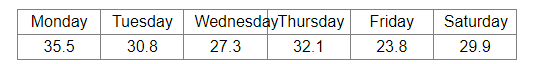# The daily minimum temperature recorded (in degree F) at a place during a week was as under:Question:

The daily minimum temperature recorded (in degree F) at a place during a week was as under:Find the mean temperature.

Solution:

Daily minimum temperatures = 35.5, 30.8, 27.3, 32.1, 23.8 and 29.9
Thus, we have:

Mean temperature $=\frac{35.5+30.8+27.3+32.1+23.8+29.9}{6}$

$=\frac{179.4}{6}$

$=29.9^{\circ} \mathrm{F}$